求一到期日提醒公式 [问题点数：100分，结帖人treesman]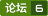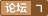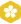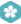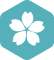2011年1月 Windows专区大版内专家分月排行榜第三
2010年11月 Windows专区大版内专家分月排行榜第三
2010年2月 Windows专区大版内专家分月排行榜第三
2010年1月 Windows专区大版内专家分月排行榜第三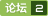2011年1月 Windows专区大版内专家分月排行榜第三
2010年11月 Windows专区大版内专家分月排行榜第三
2010年2月 Windows专区大版内专家分月排行榜第三
2010年1月 Windows专区大版内专家分月排行榜第三
1到N累加求和
DATAS SEGMENT data db 100,?,100 dup(?) ten dw 10 number dw 0 count dw 0 string db 'Error input!$' string1 db 0dh,0ah,'$' ;此处输入数据段代码 DATAS ENDS STACKS SEGMENT stack d
C程序设计 例题5.1 求一到一百的和
#include int main() { int i=1,sum=0; while(i,sum); return 0; }

#include int main() { int n,i,sun=1; printf("input n:"); scanf("%d",&n); { for (i=1;i,sun);
java求出一到一百的和
package java01; import java.util.stream.IntStream; public class Java8 { public static void main(String[] args) { //不使用循环语句，求出1+2+3.....+100的和 System.out.printf(&quot;1+2+3....+100=%d&quot;,IntStream.ra...

1. 用while循环打印九九乘法表 2.  用for循环实现九九乘法表 3.   1到10的阶乘和 一定会有更好的算法的，再想一想！

#include&lt;stdio.h&gt; int main() { double m,n,a,b=0; for(m=1;m&lt;=20;m++) {for(n=1,a=1;n&lt;=m;n++) a=a*n; b=b+a; } printf(“结果为；%f\n”,b); return 0; }

1到N的平方和公式
1²+2²+...+n²=n(n+1)(2n+1)/6 可以用数学归纳法证明 1*2 + 2*3 + 3*4 + ... + n*(n+1)  = (1²+1) + (2²+2) + (3²+3) + ... + (n²+n)  = (1²+2²+3²+...+n²) + (1+2+3+...+n)  = n(n+1)(2n+1)/6 + n(n+1)/2 = [n(n+1)/6]

#include using namespace std; int main() { int i=1,j; int sum=0,total; cout<<=i;j++) total*=j;
Java练习 输出1到10的阶乘的和 回文数 简易菜单 个人所得税

//求阶层1到10的和 //定义一个变量存放最终结果 int sum=0; //定义一个变量存放每个阶层的结果 int num=1; //定义一个循环 for (int i=1;i&amp;lt;=10;i++){ //num与i相乘得出每个阶层的结果 nu...
1到100倒数和（C）
#include&amp;lt;stdio.h&amp;gt;//1-1/2+1/3-...-1/00// void main() { double fm=1,s=0; int fh=1,fz=1; for(;fm&amp;lt;=100;fm++) { fz=fh*fz; s=s+fz/fm; fh=-fh; } ...

1到n的阶乘之和，首先我们需要在控制台输入整数 ，然后再利用循环语句求出阶乘的积并将每次的积相加即可！  写一个方法，循环求和： /** * 求1到n的阶乘和 */ public static long factorialSum(int num) { long sum = 0; long temp = 1; for (int i = 1; i &amp;lt;= nu...
C语言：一到一百阶乘
#include int n; int r={0}; void count(int n) { int i; for(i=0;i0;i--) { r[i-1]=r[i-1]+r[i]/10; r[i]=r[i]%10; } } void main() { int i; in

1到10，20到30,30到45求和
#include void sum(int begin,int end) { int i,sum=0; for(i=begin;i,begin,end,sum); } int main() { su

1. 1到n之和(利用恒等式(n+1)^2=n^2+2n+1）     (n+1)^2-n^2=2n+1,         ......（累加）        3^2-2^2=2*2+1         2^2-1^2=2*1+1.  把这n个等式两端分别相加,得：  (n+1)^2-1=2(1+2+3+...+n)+n,  整理后得：  　　1+2+3+...+n=(n+
excel到期提醒，可以用函数公式来做，颜色变化可以用条件格式来做。
excel到期<em>提醒</em>，可以用函数<em>公式</em>来做，颜色变化可以用条件格式来做。 1、函数<em>公式</em>。 如示例数据，B列分别运用了条件格式和函数<em>公式</em>，函数<em>公式</em>为：=IF(DATEDIF(A1,TODAY(),"d")=0,"已到期","未到期")，DATEDIF函数是专门来计算两个日期之间的年数、月数、天数的<em>公式</em>，这个<em>公式</em>的意思是，如果A1单元格的日期和今天相比，值等于0，就

#includeusing namespace std;int main(){{int sum=0;for(int i=1;i<<{int s
【Java】求1到100以内的素数（质数）

public class Test{    public static void main(String[] args){       int a=0;       for(int i=1;i          a+=i;       }     System.out.println(a);    } }
Java,求1到100的累加值
public class SumDemo { //1到100相加 public static void main(String[] args) { System.out.println("方法1："+sum1()); System.out.println("方法2："+sum2()); System.out.println("方法3："
【java】求1-10的和。
public class he{     public static void main(String[] args)     {    //定义变量用于存储不断变化的和;         int sum = 0;         //定义变量，记录住不断变化的被加的数；         int x = 1;         //定义循环，重复加法的过程；         while

C++语言实现1到100的偶数，奇数；（初学者）
//区别1到100的偶数与奇数 1，2,3,4,5，6.......，100 在数学中 偶数可以用2k来表示 ， 奇数用2k+1来表示。   在这里我们可以利用for循环 for （int a = 1；a {       if （a%2 == 0）                                                if是判断真假函数，此处的a%2 ==

/** *//** * FactorialOfSum.java * 求1到20的阶乘之和  * @author CodingMouse * @version 0.1 2008-4-26 */public class FactorialOfSum ...{  public static void main(String[] args) ...{  //保存当前数与上一个数阶乘的乘积结果
Java 1-100简单求和
package 简单求和; public class Simple { public static void main(String[] args) { // TODO Auto-generated method stub int num=0; for(int i=0;i&amp;lt;100;i++){ num=num+i+1; } System.out.println(...

package com.test; import java.util.*; import java.io.*; public class Main { public static void main(String[] args) { Scanner sc = new Scanner(System.in); int r1, r2, result_even,

Excel 制作生日提醒提示10天内有哪些职工快过生日

C程序设计 （第四版） 谭浩强 习题5.7 个人设计习题 5.7 求k=1，k到100的相加和，k平方到50的相加和，1/k到10的相加和。∑k=1100k+∑k=150k2+∑k=1101k\sum_{k = 1}^{100}k+\sum_{k=1}^{50}k^2+\sum_{k=1}^{10}\frac{1}{k} 代码块#include main() { doubl

1到10的阶乘和
public class Factorial{ public static void main(String [] args){ int a=1; int sum=0; for(int j=1;j<11;j++){ a=a*j; sum=a+sum; } System
java 计算当前日期所在的星期一到期日
public void mondayToSunday() { Date now = new Date(); Date time = new Date(now.getYear(), now.getMonth(), now.getDate()); SimpleDateFormat sdf = new SimpleDateFormat("yyyy-MM-dd"); // 设置时间格式 C
C语言基础之求1到20的阶乘

DATA SEGMENT TS DB 0 HD DB 0 TE DB 0 BI DB 0 DATA ENDS CODE SEGMENT ASSUME CS:CODE,DS:DATA START: MOV AX,DATA MOV DS,AX MOV CX,100 MOV AX,0 SIGN: MOV BX,CX TEST BX,01H JZ ADD_...

# -*- coding:cp936 -*- #作者 zyc #2015/11/9 #计算1~10阶乘然后累加 #2015/11/11完成 print "计算1~10阶乘然后累加" str1 = "" sum1 = 1 sum2 = 0 for j in range(1,11): for i in range(1, j): sum1 = sum1 * i sum2

1到100以内所有奇数的和
/** ***计算1到100以内所有奇数的和的程序 */ public class OddSum{ public static void main(String args[]){ int result = 0 ； for(int i = 1 ; i result +=i ; } System.out.println("result = " + result);
MATLAB求圆心坐标公式代码
MATLAB求圆心坐标<em>公式</em>代码

python 1加到一百

POI 导入Excel 提醒LeftoverDataException求帮助
POI 导入Excel <em>提醒</em>LeftoverDataException求帮助
C程序设计 例题5.2 用do...while语句求一到一百的和
#includeint main(){int i=1,sum=0;do{sum=sum+i;i++;}while(i,sum);return 0;}

public static void main(String[] args) { for (int i = 2; i j) { if (i % j == 0) { m++; } } ...

﻿﻿ 很短小精悍了，一个for循环实现：

ros到期三天<em>提醒</em>

/**  * FactorialOfSum.java  * 求1到20的阶乘之和   * @author CodingMouse  * @version 0.1 2008-4-26  */ public class FactorialOfSum {    public static void main(String[] args) {   //保存当前数与上一个数阶乘的乘积结

C语言求：1到100之间的所有素数之和
1到100之间的所有素数之和；素数曾称质数。一个大于1的正整数，如果除了1和它本身以外，不能被其他正整数整除，就叫素数。1不是素数。 2+3+5+7+11+13+17+19+23+29+31+37+41+43+47+53+59+61+67+71+73+79+83+89+97=1060, 答:100以内所有素数之和是1060 #include &quot;stdafx.h&quot; #include&amp;lt;s...

#include &lt;stdio.h&gt; /*<em>一到</em>二十阶乘求和*/ int main(){ int t; int m,i; int sum=0; for(i=1;i&lt;=20;i++) { for(m=1,t=1;m&lt;=i;m++) { t=t*m; } sum=sum+t; } printf("%d",sum); return 0; }...

VC++求n的阶乘和
int n,i=1;long sum=0,s=1; scanf("%d",&n); while(i<=n)
python--递归计算n的价乘
#计算输入的任意N的阶乘 方法一 import sys """print("---------方法一 ---------") def func_one(n):     tmp = 1     for i in range(1,n+1):         tmp *= i         print(i)     return tmp re = int(input("请输入您要

int i=1,sum=0;   do{    sum+=i;    i++;   }while(i   System.out.println("1+2+3+..+10="+sum);

Private Sub Command7_Click() Cls Print f(10) End Sub Private Function g(x As Integer) As Long If x = 1 Then g = x Else g = x + g(x - 1) End If End Function

/* * 程序的版权和版本声明部分: * Copyright (c) 2011, 烟台大学计算机学院 * All rights reserved. * 文件名称：test.cpp * 作 者：初瑞 * 完成日期：2013 年 11 月 22 日 * 版 本 号：v1.0 * 对任务及求解方法的描述部分: * 输入描述：7 * 问题描述：求奇数连乘的积 * 程序输出：10

1到10所有数的立方
#include main() { int i,n; long p=1; printf("please enter n:"); scanf("%d",&n); for(i=1;i { p=i*i*i; printf("%d=%1d\n",i,p); } }

#include int main() {     int n, i, n1 = 1,s=0,m;     scanf("%d",&m);     for(int j=1;j<= n; i ++)     {         n1*=i;
C经典算法之最大公因数、最小公倍数、因式分解

public class T0302{ public static void main(String args[]){ int a = 5; int temp = array(a); System.out.println(temp); } public static int array(int n){ int sum = 0; for(int
1到100的和（C）
#include&amp;lt;stdio.h&amp;gt;//1到100和// void main() { int i,s; s=0; for(i=1;i&amp;lt;=100;i++) { s=s+i; } printf (“s=%d\n”,s); }

while循坏1到100的和
-
C 1到n求和问题，n的最大值，及函数递归调用求和
S=1+2+3+…+n; #include &amp;lt;stdio.h&amp;gt; main() { unsigned long i,n,s=0; scanf(&quot;%u&quot;, &amp;amp;n); for(i=0;i&amp;lt;=n;i++) s+=i; printf(&quot;%u&quot;, s); } 把变量i,n,s定义为无符号长整型，n最大值为92681 也可以把变量i,n,s定义为单精度实型， scanf(&quot;%f&quot;, &amp;...
Java求1-100之和的三种方式
java计算1到100之和
1到10之间所有数的平方和立方
#include main() { int i,n; long p=1,j=1; printf("please enter n:"); scanf("%d",&n); for(i=1;i { p=i*i; printf("%d=%1d\n",i,p); } for(i=1;i { j=i*i*i; printf("%d=%1d\n",i,j); } }
C++函数作业参考答案

C++程序设计函数作业

C语言求1到20的阶乘的和

1*3*…*n奇数乘积
﻿﻿ #include long f(int); int main( ) {     int n;     long y;     printf("请输入一个数 ：");     scanf("%d", &n);     if(n%2) //若奇数         y=f(n);     else         y=f(n-1);     printf("%d以内的奇

STACK SEGMENT STACK    DB 200 DUP(0)STACK ENDSDATA SEGMENT SUM DW ? DATA ENDS CODE SEGMENT  ASSUME CS:CODE,DS:DATA,SS:STACK BEGIN: MOV AX,DATA     MOV DS,AX     MOV AX,0     MOV BX,1     MOV CX,50 N

Eclipse快捷键大全.pdf下载

WCDMA天线原理与应用下载